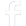#### Connect To Facebook#### WhatsApp Quick Chat#### Get Instant Call Back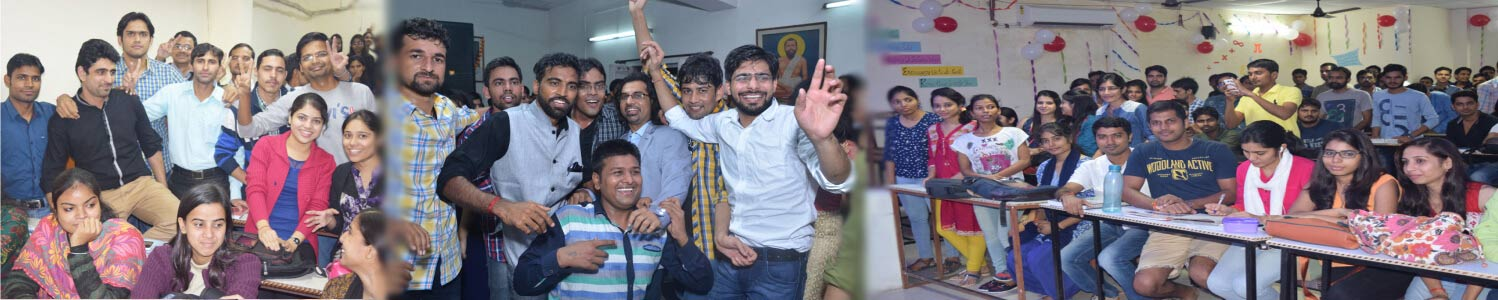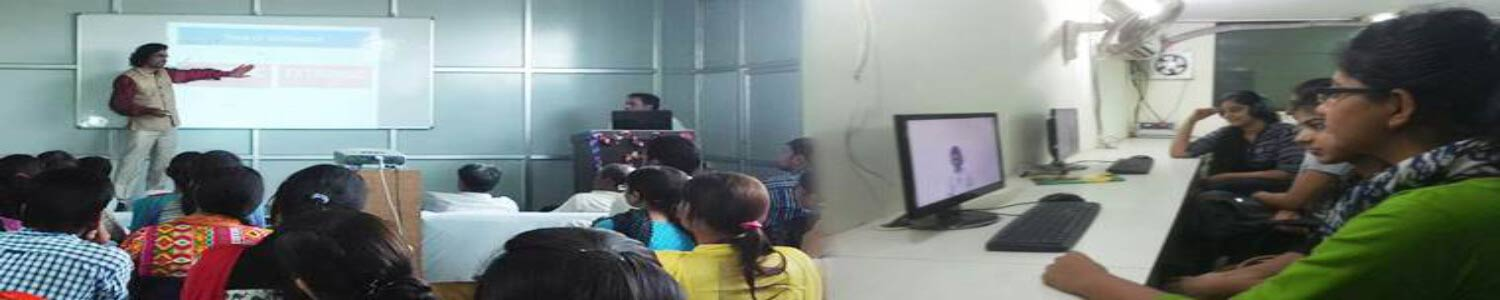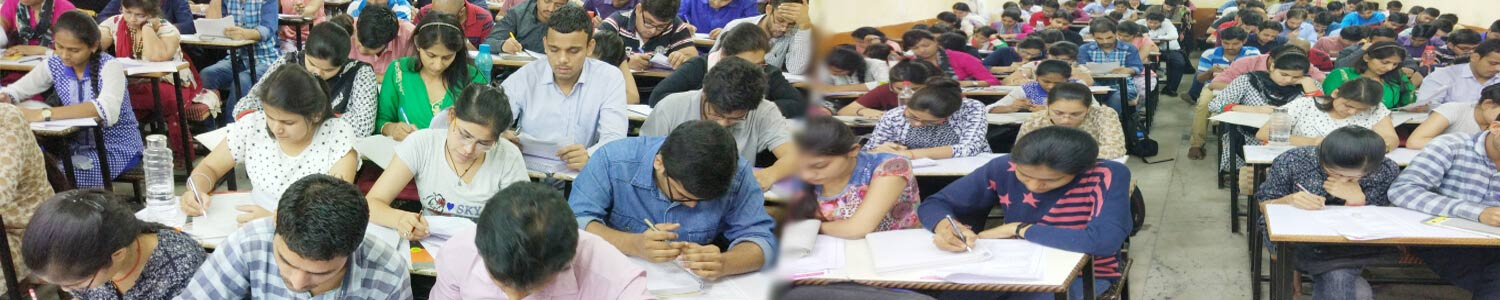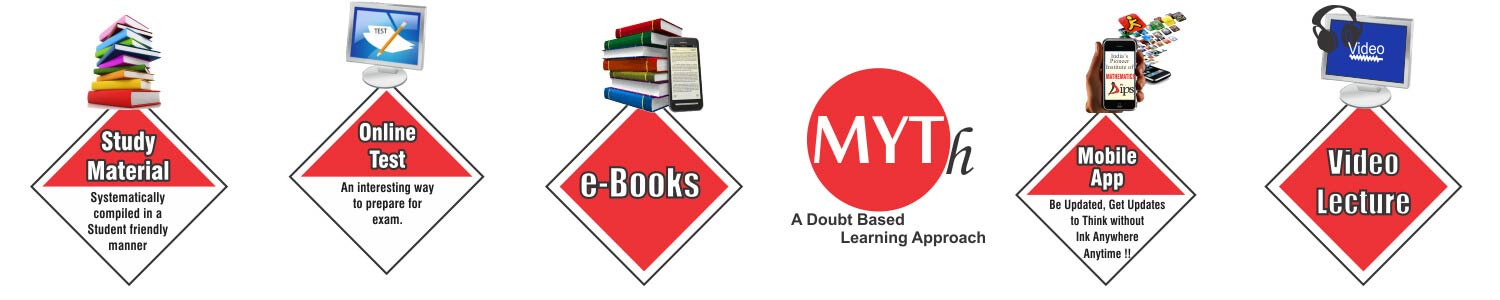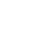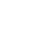## Offers## News & Events#### Syllabus and reference Book

##### Linear Algebra:

Finite dimensional vector spaces; Linear transformations and their matrix representations, rank; systems of linear equations, eigen values and eigen vectors, minimal polynomial, Cayley-Hamilton Theroem, diagonalisation, Hermitian, Skew-Hermitian and unitary matrices; Finite dimensional inner product spaces, Gram-Schmidt orthonormalization process, self-adjoint operators.

##### Book Recommendations:

Linear Algebra - Schaum's Series - (Tata McGraw-Hill Publication)
Linear Algebra - Kenneth M Hoffman Ray Kunzet (PHI Publication)
Linear Algebra - Vivek Sahai, Vikas Bist (Narosa Publication)

##### Complex Analysis

Algebra of complex numbers, the complex plane, polynomials, power series, transcendental functions such as exponential, trigonometric and hyperbolic functions. Analytic functions, Cauchy-Riemann equations. Contour integral, Cauchy's theorem, Cauchy's integral formula, Liouville's theorem, Maximum modulus principle, Schwarz lemma, Open mapping theorem. Taylor series, Laurent series, calculus of residues. Conformal mappings, Mobius transformations.

Complex Variables - H.S. Kasana (PHI Publication)
Complex Analysis - S Ponnusamy (Narosa Publication)
Complex Analysis - R.V. Churchill (Tata McGraw-Hill Publication)

Real Analysis: Sequences and series of functions, uniform convergence, power series, Fourier series, functions of several variables, maxima, minima; Riemann integration, multiple integrals, line, surface and volume integrals, theorems of Green, Stokes and Gauss; metric spaces, completeness, Weierstrass approximation theorem, compactness; Lebesgue measure, measurable functions; Lebesgue integral, Fatou's lemma, dominated convergence theorem.

Principle of Mathematical Analysis - S.L. Gupta, N.R. Gupta (Pearson Publication)
Real Analysis - Robert G Bartle (Wiley Publication)
Real Analysis - M.D. Raisinghania (S. Chand Publication)

Ordinary Differential Equations: First order ordinary differential equations, existence and uniqueness theorems, systems of linear first order ordinary differential equations, linear ordinary differential equations of higher order with constant coefficients; linear second order ordinary differential equations with variable coefficients; method of Laplace transforms for solving ordinary differential equations, series solutions; Legendre and Bessel functions and their orthogonality.

Topology - K. D. Joshi (New Age International Publication)

##### ORDINARY DIFFERENTIAL EQUATIONS (ODE)

Existence and uniqueness of solutions of initial value problems for first order ordinary differential equations, singular solutions of first order ODE , system of first order ODE. General theory of homogenous and non-homogeneous linear ODEs, variation of parameters, Sturm-Liouville boundary value problem, Green's function.

Ordinary Differential Equations - MD Rai Singhania ( S.Chand Publication)
Differential Equations - Shepley L. Ros (Wiley Publication)
Differential Equations - Earl Codington (PHI Publication)

Algebra: Normal subgroups and homomorphism theorems, automorphisms; Group actions, Sylow's theorems and their applications; Euclidean domains, Principle ideal domains and unique factorization domains. Prime ideals and maximal ideals in commutative rings; Fields, finite fields.

Contemporary Abstract Algebra - Joseph A. Gallian (Narosa Publication)
Modren Algebra - Surjeet Singh and Qazi Zameeruddin (Vikas Publication)

Functional Analysis: Banach spaces, Hahn-Banach extension theorem, open mapping and closed graph theorems, principle of uniform boundedness; Hilbert spaces, orthonormal bases, Riesz representation theorem, bounded linear operators.

Numerical Analysis: Numerical solution of algebraic and transcendental equations: bisection, secant method, Newton-Raphson method, fixed point iteration; interpolation: error of polynomial interpolation, Lagrange, Newton interpolations; numerical differentiation; numerical integration: Trapezoidal and Simpson rules, Gauss 51 Legendre quadrature, method of undetermined parameters; least square polynomial approximation; numerical solution of systems of linear equations: direct methods (Gauss elimination, LU decomposition); iterative methods (Jacobi and Gauss-Seidel); matrix eigenvalue problems: power method, numerical solution of ordinary differential equations: initial value problems: Taylor series methods, Euler's method, Runge-Kutta methods.

Numerical Analysis - R.K. Jain, S.R.K. Iyengar (New Age Publication)

Partial Differential Equations: Linear and quasilinear first order partial differential equations, method of characteristics; second order linear equations in two variables and their classification; Cauchy, Dirichlet and Neumann problems; solutions of Laplace, wave and diffusion equations in two variables; Fourier series and Fourier transform and Laplace transform methods of solutions for the above equations.

Partial Differential Equations - T. Amaranath (Narosa Publication)
Introduction To Partial Differential Equations - K. Sankara Rao (PHI Publication)

Mechanics: Virtual work, Lagrange's equations for holonomic systems, Hamiltonian equations.

Topology: Basic concepts of topology, product topology, connectedness, compactness, countability and separation axioms, Urysohn's Lemma.

Topology - K. D. Joshi (New Age International Publication)

Probability and Statistics: Probability space, conditional probability, Bayes theorem, independence, Random variables, joint and conditional distributions, standard probability distributions and their properties, expectation, conditional expectation, moments; Weak and strong law of large numbers, central limit theorem; Sampling distributions, UMVU estimators, maximum likelihood estimators, Testing of hypotheses, standard parametric tests based on normal, X2 , t, F – distributions; Linear regression; Interval estimation.

Probablity and statistics - S. C. Gupta and ‎V. K. Kapoor (S.Chand Publication)

## Offers